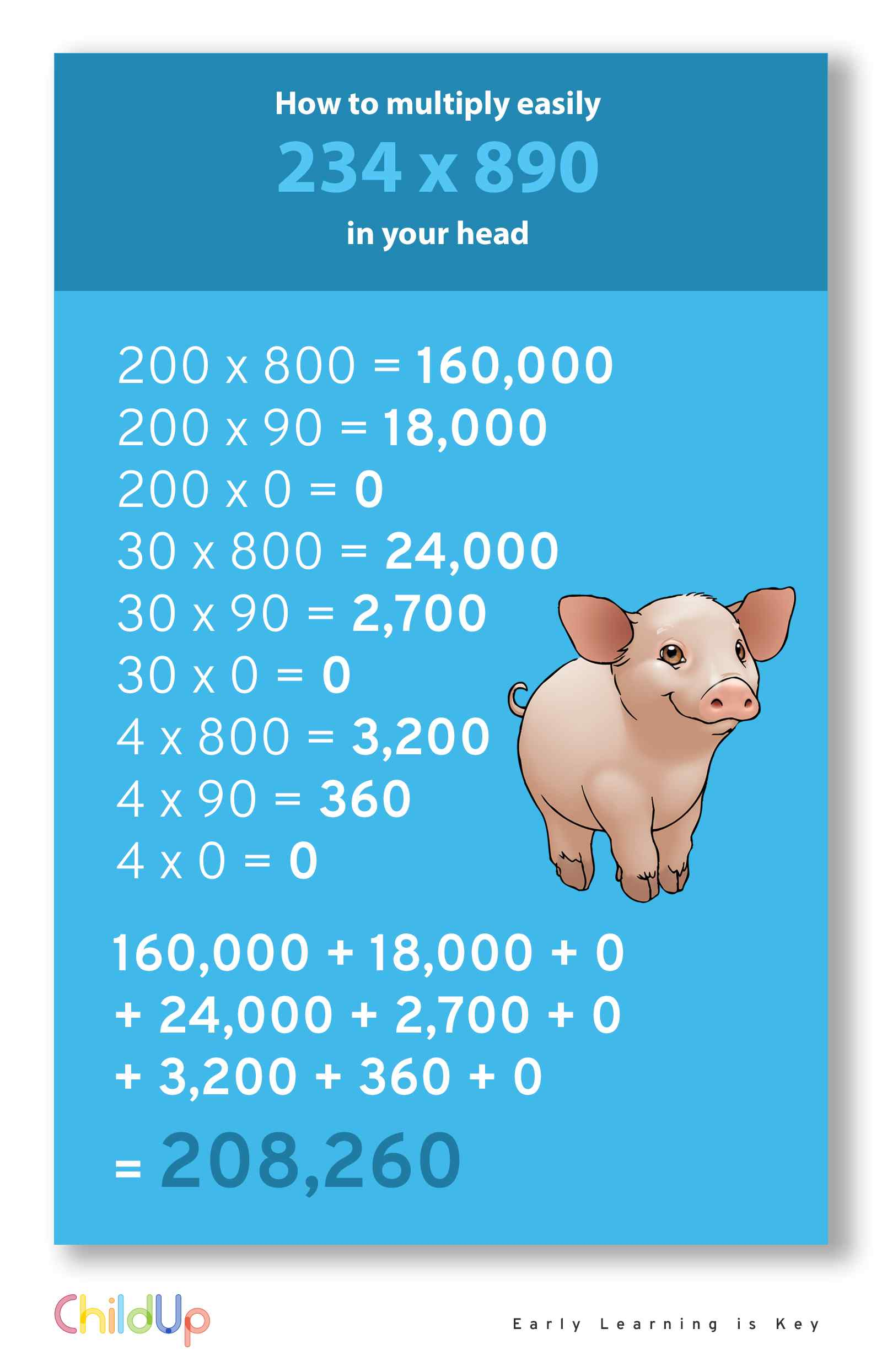How to multiply easily two 3-digt numbers in your head, for example 234 x 890:

200 x 800 = 160,000
200 x 90 = 18,000
200 x 0 = 0
30 x 800 = 24,000
30 x 90 = 2,700
30 x 0 = 0
4 x 800 = 3,200
4 x 90 = 360
4 x 0 = 0

160,000 + 18,000 + 0 + 24,000 + 2,700 + 0 + 3,200 + 360 + 0 = 208,260